# RS Aggarwal Class 7 Solutions Chapter 4 Rational Numbers Ex 4B

In this chapter, we provide RS Aggarwal Solutions for Class 7 Chapter 4 Rational Numbers Ex 4B for English medium students, Which will very helpful for every student in their exams. Students can download the latest RS Aggarwal Solutions for Class 7 Chapter 4 Rational Numbers Ex 4B Maths pdf, free RS Aggarwal Solutions Class 7 Chapter 4 Rational Numbers Ex 4B Maths book pdf download. Now you will get step by step solution to each question.

### RS Aggarwal Solutions for Class 7 Chapter 4 Rational Numbers Ex 4B Download PDF

Question 1.
Solution:
(i) Draw a number line and locate a point O on it. Let it represent 0 Now 13 has been presented on the number line given below.(ii) Draw a number line and locate a point O on it. Let it represent 0. The number 27 has been represented on the number line given below: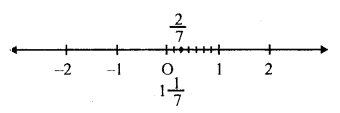(iii) Draw a number line and locate a point O on it. Let it represent 0. The number 73 has been represented on the number line given below:(iv) Draw a number line and locate a point O on it. Let it represent 0. The number 73 has been represented on it as given below:(v) Draw a number line and locate a point O on it. Let it represented 0. The number 378 has been represented on it as given below:
378 = 458(vi) Draw a number line and locate a point O on it. Let it represent 0. The number −13 has been represented on it as given below: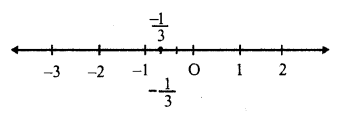(vii) Draw a number line and locate a point O on it. Let it represent 0. The number −34 has been represented on it is as given below: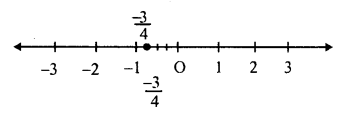(viii) Draw a number line and locate a point on it. Let it represent 0. The number −127 has been represented on it as given below:(ix) Draw a number line and locate a point O on it. Let it represent 0. The number 36−5 has been represented on it as given below:(x) Draw a number line and locate is point O on it. Let is represent 0. The number −439 has been represented on it as given below:Question 2.
Solution:
(i) 56 or 0, 56 is greater as any positive number is always greater than 0.Question 3.
Solution:Question 4.
Solution:Question 5.
Solution:Question 6.
Solution: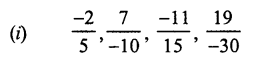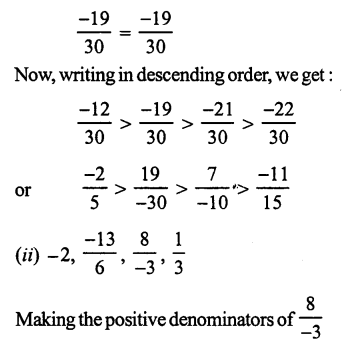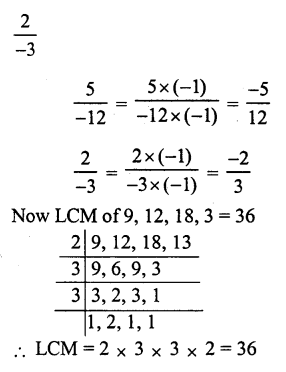Question 7.
Solution:
(i) True: All negative numbers lie on the left of 0.
(ii) False: All negative numbers lie on the left of 0.
(iii) True: All positive numbers lie on the right of 0 and all negative numbers on the left of 0.
(iv) False: −18−13 = 1813 which is positive and positive number lie on the left of 0.
(v) True: −5−8 = 58 which is positive and all positive number lie on the right of negative numbers.
(i), (iii) and (iv) are true.

Question 8.
Solution:
5 rational numbers between -3 and -2.Question 9.
Solution: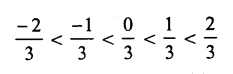Question 10.
Solution:
L.C.M. of 5 and 2 = 10All Chapter RS Aggarwal Solutions For Class 7 Maths

—————————————————————————–

All Subject NCERT Exemplar Problems Solutions For Class 7

All Subject NCERT Solutions For Class 7

*************************************************

I think you got complete solutions for this chapter. If You have any queries regarding this chapter, please comment on the below section our subject teacher will answer you. We tried our best to give complete solutions so you got good marks in your exam.

If these solutions have helped you, you can also share rsaggarwalsolutions.in to your friends.